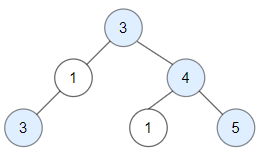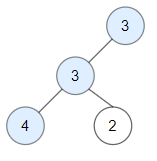# 1448. Count Good Nodes in Binary Tree

https://leetcode.com/problems/count-good-nodes-in-binary-tree/

Given a binary tree root, a node X in the tree is named good if in the path from root to X there are no nodes with a value greater than X. Return the number of good nodes in the binary tree.

Example 1:Input: root = [3,1,4,3,null,1,5] Output: 4 Explanation: Nodes in blue are good. Root Node (3) is always a good node. Node 4 -> (3,4) is the maximum value in the path starting from the root. Node 5 -> (3,4,5) is the maximum value in the path Node 3 -> (3,1,3) is the maximum value in the path. Example 2:Input: root = [3,3,null,4,2] Output: 3 Explanation: Node 2 -> (3, 3, 2) is not good, because “3” is higher than it. Example 3: Input: root =  Output: 1 Explanation: Root is considered as good.

Constraints:

``````The number of nodes in the binary tree is in the range [1, 10^5].
Each node's value is between [-10^4, 10^4].
``````

• code
``````class Solution {
private int result = 0;
public int goodNodes(TreeNode root) {
dfs(root, Integer.MIN_VALUE);
return result;
}

private void dfs(TreeNode node, int curMax){
if (node == null) return;
if (node.val >= curMax) result++;
dfs(node.left, Math.max(curMax, node.val));
dfs(node.right, Math.max(curMax, node.val));
}
}
``````